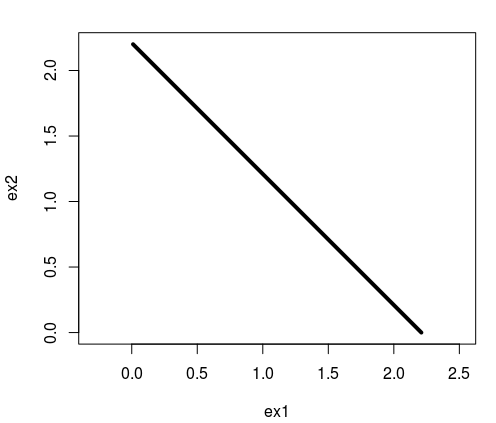# Expected goals from over/under odds

I got a comment the other day asking about whether it is possible to get the expected number of goals scored from over/under odds, similar to how you can do this for odds for win, draw or lose outcomes. The over/under odds refer to the odds for the total score (the sum of the score for two opponents) being over or under a certain value, usually 2.5 in soccer.

It is possible, and rather easy even, to get the expected total score from the over/under odds, at least if you assume that the number of goals scored by the two teams follows a Poisson distribution. This is the same assumption that makes the method for extracting the expected goals from HDW odds possible. The Poisson distribution is really convenient and reasonable realistic probability model for different scorelines. It is controlled by a single parameter, called lambda, that is also the expected value (and the expected goals in this case). One convenient property of the Poisson is that the sum of two Poisson distributed variables with parameters lambda1 and lambda2 is also Poisson distributed, with the lambda being the sum of the two lambdas, i.e. lambdasum = lambda1 + lambda2.

So how can you find the expected total number of goals based on the over/under odds? First you need to convert the odds for the under outcome to a proper probability. How you do this depends on the format your odds come in, but in R you can use the odds.converter package to convert them to decimal format, and then use my own package called implied to convert them to proper probabilities.

After you have the probabilities for the under probability, you can use the Poisson formula to find the value of the parameter lambda that gives the probability for the under outcome that matched the probability from the odds. In R you can use the built-in ppois function to compute the probabilities for there being scored less than 2.5 goals when the expected total goals is 3.1 like this:

```under <- 2.5
ppois(floor(under), lambda=3.1)
```

This will give us that the probability is 40.1% of two or less goals being scored in total, when the expected total is 3.1. Now you can try to manually adjust the lambda parameter until the output matches your probability from the odds. Another way is to automate this search using the built-in uniroot function. The uniroot function takes as input another function, and searches for the input value that gives the result 0. We therefore have to write a function that takes as input the expected goals, the probability implied by the odds, and the over/under limit, and returns the difference between the probability from the Poisson model and the odds probability. Here is one such function:

```obj <- function(expg, under_prob, under){
(ppois(floor(under), lambda=expg) - under_prob)
}
```

Next we feed this to the uniroot function, and gives a realistic search interval for the expected goals, between 0.01 and 10 in this case, and the rest of the parameters. For this example I used 62% chance of there being scored less than 2.5 goals.

```uniroot(f = obj,
interval = c(0.01, 10),
under_prob = 0.62,
under = 2.5)
```

From this I get that the expected total goals is 2.21.

You might wonder if it is possible to get the separate expected goals for the two teams from over/under odds using this method. This is unfortunately not possible. The only thing you can hope for is to get a range of possible values for the two expected goals that sums to the total expected goals. In our example with the expected total goals being 2.21, the range of possible values for the two expected goals can be plotted as a line like this:If course, you can judge some pairs of expected goals being more likely than others, but there is no information about this in over/under odds alone. It might be possible, I am not 100% sure, that other non-Poisson models, which would involve more assumptions, could exploit the over/under odds to get expected goals for both teams.